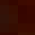## Tuesday, 4 December 2012

### Class 7 - NSO Test Paper - Mental Ability (2012)

NSO Test Paper -  (2012)
Mental Ability

Q1: Which of the following statements is INCORRECT?

(a) All integers and fractions are called rational numbers.
(b) The number 0 is neither a positive nor a negative rational number.
(c) There are limited number of rational numbers between two rational numbers.
(d) A number that can be expressed in the form of p/q, where p and q are integers and q ≠0 is called rational number.

Q2: Aman rotated a game piece 270° clock wise around vertex V as shown in figure. Which of the following represent the position of the game piece after 270° clock wise rotation?

Q3: Lengths of some world's longest snakes are 9, 9, 4.3, 6, 6.5, 2.6, 5.6, 9.
The range in metres of the lengths is _________.

(a) 6.25
(b) 6.4
(c) 6.5
(d) 9

Q4: The least number which when increased by 5 is divisible by each one of 24, 32, 36 and 54 is______.

(a) 427
(b) 859
(c) 869
(d) 4320

Q5:Amit, Raj, Kunal, Harsh and Armaan used different types of transport during holidays.
1. Everyone except Harsh travelled on the bus.
2. Kunal, Harsh and Armaan all went on an aeroplane.
3. Everyone except Raj went in a taxi.
4. Amit and Harsh both had a ride on a tram.
5. Raj travelled on a bus and then a train to get his holiday destination.
Who used the fewest types of transport during holidays?

(a) Amit
(b) Raj
(c) Harsh
(d) Armaan

Q6: Solve for x:

` x + 3   x + 4    x + 5    x + 6`
`  ̶ ̶ ̶ ̶ ̶ -  ̶ ̶ ̶ ̶ ̶ =  ̶ ̶ ̶ ̶ ̶ ̶  -  ̶ ̶ ̶ ̶ ̶ `
`   4       5       6         7`

(a) -1
(b) 1
(c) 0
(d) 2

Q7: 60% of the population of city were men and the rests were women. Afterwards, 840 men left the city and 1000 women came to the city. As a result, the percentage of the men reduced to 56% of the population. The present population of the city is ________.

(a) 23420
(b) 24300
(c) 23240
(d) 23400

Q8: The given table shows a relationship between x and y.

 x 0 2 4 6 8 y 2 9 16 23 30

The equation which is true for each of the ordered pairs in the table is ______.

(a) y = 3x + 2
(b) y = 2.5x + 6
(c) y = 3.5x + 2
(d) y = 3x + 6

Q9: The records of  a sporting goods company shows that 4 out of every 100 footballs manufactured have some defect. The probability of drawing a football that will not have a manufacturing defect is _______.

(a) 1/1
(b) 1/4
(c) 1/25
(d) 24/25

Q10: In what time will the interest on a certain sum of money become (3/8)th of the amount at the rate of 6% p.a simple interest.

(a) 5 years
(b) 10 years
(c) 8 years
(d) 6 years

Q11: In the problem set, given below three figures are similar in a certain manner. However one figure is not like the other three. Choose the figure which is different from the rest.

Q12: If
`     1     1          4`
`    8 ̶ ̶ - 4 ̶ ̶ + 2.8 +  ̶ ̶ - 2.32 = 5.33`
`     4     5          x `
then x is _________.

(a) 0.05
(b) 0.5
(c) 5
(d) none of these

Q13: Select the figure which is embedded in the given figure (X).

Q14: Which of the following numbers is divisible by 9?

(a) 823519
(b) 270351
(c) 612593
(d) 759803

Q15: The elevator descends into a mineshaft at the rate of 8m/min. If the descent starts from 20m above the ground level, the time taken by the elevator to reach 300 m is

(a) 30 mins
(b) 40 mins
(c) 50 mins
(d) 1 hr1.